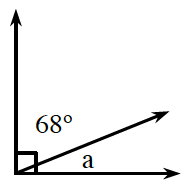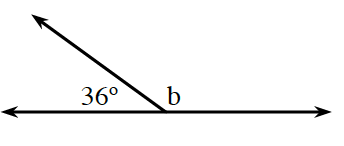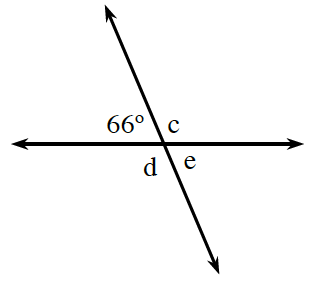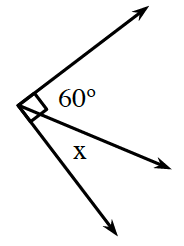### Home > ACC7 > Chapter 9 Unit 6 > Lesson CC2: 9.1.2 > Problem9-34

9-34.

For each diagram below, identify each set of angles as complementary, supplementary, or vertical. Then use what you know about the properties of angles to find the measure of each angle labeled with a variable.

1.Write an equation relating $68°$ and $a$.
$68°+a=90°$

Subtract $68°$ from both sides.
$a=90°-68°=22°$

$22°$; they are complementary angles

1.These angles are supplementary, so $36°+b=180°$. What is the measure of $b$?

1.e and $66°$ are vertical angles, so they are equal in measure. $d$ and $c$ are also vertical angles.

Angle $c$ is $114°$. Use this to help you find $d$ and $e$.

1.